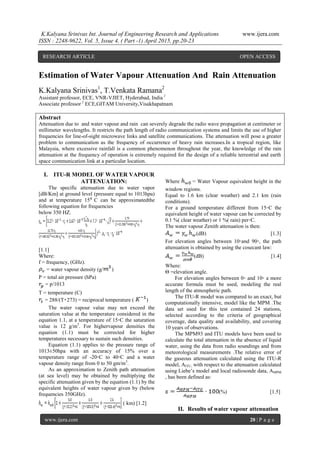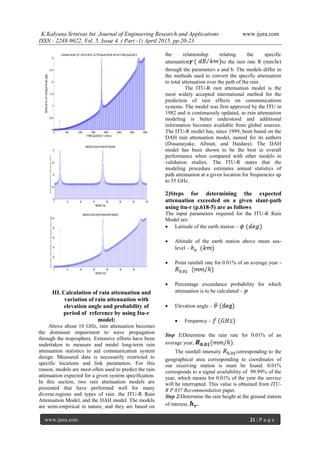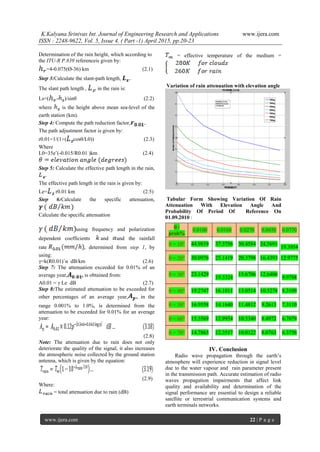Seu SlideShare está sendo baixado. ×

# Estimation of Water Vapour Attenuation And Rain Attenuation

Anúncio
Anúncio
Anúncio
Anúncio
Anúncio
Anúncio
Anúncio
Anúncio
Anúncio
Anúncio
AnúncioCarregando em…3
×

1 de 4 Anúncio

# Estimation of Water Vapour Attenuation And Rain Attenuation

Attenuation due to and water vapour and rain can severely degrade the radio wave propagation at centimeter or millimeter wavelengths. It restricts the path length of radio communication systems and limits the use of higher frequencies for line-of-sight microwave links and satellite communications. The attenuation will pose a greater problem to communication as the frequency of occurrence of heavy rain increases.In a tropical region, like Malaysia, where excessive rainfall is a common phenomenon throughout the year, the knowledge of the rain attenuation at the frequency of operation is extremely required for the design of a reliable terrestrial and earth space communication link at a particular location.

Attenuation due to and water vapour and rain can severely degrade the radio wave propagation at centimeter or millimeter wavelengths. It restricts the path length of radio communication systems and limits the use of higher frequencies for line-of-sight microwave links and satellite communications. The attenuation will pose a greater problem to communication as the frequency of occurrence of heavy rain increases.In a tropical region, like Malaysia, where excessive rainfall is a common phenomenon throughout the year, the knowledge of the rain attenuation at the frequency of operation is extremely required for the design of a reliable terrestrial and earth space communication link at a particular location.

Anúncio
Anúncio

### Estimation of Water Vapour Attenuation And Rain Attenuation

1. 1. K.Kalyana Srinivas Int. Journal of Engineering Research and Applications www.ijera.com ISSN : 2248-9622, Vol. 5, Issue 4, ( Part -1) April 2015, pp.20-23 www.ijera.com 20 | P a g e Estimation of Water Vapour Attenuation And Rain Attenuation K.Kalyana Srinivas1 , T.Venkata Ramana2 Assistant professor, ECE, VNR-VJIET, Hyderabad, India 1 Associate professor 2 ECE,GITAM University,Visakhapatnam Abstract Attenuation due to and water vapour and rain can severely degrade the radio wave propagation at centimeter or millimeter wavelengths. It restricts the path length of radio communication systems and limits the use of higher frequencies for line-of-sight microwave links and satellite communications. The attenuation will pose a greater problem to communication as the frequency of occurrence of heavy rain increases.In a tropical region, like Malaysia, where excessive rainfall is a common phenomenon throughout the year, the knowledge of the rain attenuation at the frequency of operation is extremely required for the design of a reliable terrestrial and earth space communication link at a particular location. I. ITU-R MODEL OF WATER VAPOUR ATTENUATION: The specific attenuation due to water vapor [dB/Km] at ground level (pressure equal to 1013hpa) and at temperature 15⁰ C can be approximatedthe following equation for frequencies below 350 HZ. [1.1] Where: f = frequency, (GHz). = water vapour density (g/ ) P = total air pressure (hPa) = p/1013 T = temperature (C) = 288/(T+273) = reciprocal temperature ( ) The water vapour value may not exceed the saturation value at the temperature considered in the equation 1.1, at a temperature of 15◦C the saturation value is 12 g/m3 . For highervapour densities the equation (1.1) must be corrected for higher temperatures necessary to sustain such densities. Equation (1.1) applies to the pressure range of 1013±50hpa with an accuracy of 15% over a temperature range of -20◦C to 40◦C and a water vapour density range from 0 to 50 gm/m3 . As an approximation to Zenith path attenuation (at sea level) may be obtained by multiplying the specific attenuation given by the equation (1.1) by the equivalent heights of water vapour given by (below frequencies 350GHz). ( km) [1.2] Where = Water Vapour equivalent height in the window regions. Equal to 1.6 km (clear weather) and 2.1 km (rain conditions). For a ground temperature different from 15◦C the equivalent height of water vapour can be corrected by 0.1 %( clear weather) or 1 %( rain) per◦C. The water vapour Zenith attenuation is then: (dB) [1.3] For elevation angles between 10◦and 90◦, the path attenuation is obtained by using the cosecant law: (dB) [1.4] Where: Θ =elevation angle. For elevation angles between 0◦ and 10◦ a more accurate formula must be used, modeling the real length of the atmospheric path. The ITU-R model was compared to an exact, but computationally intensive, model like the MPM .The data set used for this test contained 24 stations, selected according to the criteria of geographical coverage, data quality and availability, and covering 10 years of observations. The MPM93 and ITU models have been used to calculate the total attenuation in the absence of liquid water, using the data from radio soundings and from meteorological measurements .The relative error of the gaseous attenuation calculated using the ITU-R model, AITU, with respect to the attenuation calculated using Liebe’s model and local radiosonde data, AMPM , has been defined as: (%) [1.5] II. Results of water vapour attenuation RESEARCH ARTICLE OPEN ACCESS
2. 2. K.Kalyana Srinivas Int. Journal of Engineering Research and Applications www.ijera.com ISSN : 2248-9622, Vol. 5, Issue 4, ( Part -1) April 2015, pp.20-23 www.ijera.com 21 | P a g e 0 50 100 150 200 250 300 350 0 0.5 1 1.5 2 2.5 3 FREQUENCY (GHz) SPECIFICATTENUATION(dB) VARIATION OF SPECIFIC ATTENUATION WITH FREQUENCY 0 50 100 150 200 250 300 350 33 33.5 34 34.5 35 FREQUENCY(GHz) EQUIVALENTHEIGHT(Km) VARIATIONOFEQUIVALENTHEIGHTWITHFREQUENCY 0 50 100 150 200 250 300 0 20 40 60 80 100 FREQUENCY(GHZ) WATERVAPOURATTENUATION(dB) VARIATIONOFWATERVAPOURATTENUATIONWITHFREQUENCY III. Calculation of rain attenuation and variation of rain attenuation with elevation angle and probability of period of reference by using Itu-r model: Above about 10 GHz, rain attenuation becomes the dominant impairment to wave propagation through the troposphere. Extensive efforts have been undertaken to measure and model long-term rain attenuation statistics to aid communication system design. Measured data is necessarily restricted to specific locations and link parameters. For this reason, models are most often used to predict the rain attenuation expected for a given system specification. In this section, two rain attenuation models are presented that have performed well for many diverse.regions and types of rain: the ITU-R Rain Attenuation Model, and the DAH model. The models are semi-empirical in nature, and they are based on the relationship relating the specific attenuation to the rain rate R (mm/hr) through the parameters a and b. The models differ in the methods used to convert the specific attenuation to total attenuation over the path of the rain. The ITU-R rain attenuation model is the most widely accepted international method for the prediction of rain effects on communications systems. The model was first approved by the ITU in 1982 and is continuously updated, as rain attenuation modeling is better understood and additional information becomes available from global sources. The ITU-R model has, since 1999, been based on the DAH rain attenuation model, named for its authors (Dissanayake, Allnutt, and Haidara). The DAH model has been shown to be the best in overall performance when compared with other models in validation studies. The ITU-R states that the modeling procedure estimates annual statistics of path attenuation at a given location for frequencies up to 55 GHz. 2)Steps for determining the expected attenuation exceeded on a given slant-path using itu-r (p.618-5) are as follows The input parameters required for the ITU-R Rain Model are:  Latitude of the earth station –  Altitude of the earth station above mean sea- level –  Point rainfall rate for 0.01% of an average year -  Percentage exceedance probability for which attenuation is to be calculated –  Elevation angle –  Frequency – Step 1:Determine the rain rate for 0.01% of an average year, . The rainfall intensity corresponding to the geographical area corresponding to coordinates of our receiving station is must be found. 0.01% corresponds to a signal availability of 99.99% of the year, which means for 0.01% of the year the service will be interrupted. This value is obtained from ITU- R P.837 Recommendation paper. Step 2:Determine the rain height at the ground station of interest, .
3. 3. K.Kalyana Srinivas Int. Journal of Engineering Research and Applications www.ijera.com ISSN : 2248-9622, Vol. 5, Issue 4, ( Part -1) April 2015, pp.20-23 www.ijera.com 22 | P a g e Determination of the rain height, which according to the ITU-R P.839 referenceis given by: =4-0.075(Ø-36) km (2.1) Step 3:Calculate the slant-path length, The slant path length , , in the rain is: Ls=( - )/sinθ (2.2) where is the height above mean sea-level of the earth station (km). Step 4: Compute the path reduction factor, The path adjustment factor is given by: r0.01=1/(1+( cosθ/L0)) (2.3) Where L0=35eˆ(-0.015/R0.01 )km (2.4) Step 5: Calculate the effective path length in the rain, The effective path length in the rain is given by: Le= r0.01 km (2.5) Step 6:Calculate the specific attenuation, Calculate the specific attenuation using frequency and polarization dependent coefficients and and the rainfall rate , determined from step 1, by using: γ=k(R0.01)ˆα dB/km (2.6) Step 7: The attenuation exceeded for 0.01% of an average year, , is obtained from: A0.01 = γ Le dB (2.7) Step 8:The estimated attenuation to be exceeded for other percentages of an average year, , in the range 0.001% to 1.0%, is determined from the attenuation to be exceeded for 0.01% for an average year: (2.8) Note: The attenuation due to rain does not only deteriorate the quality of the signal, it also increases the atmospheric noise collected by the ground station antenna, which is given by the equation: (2.9) Where: = total attenuation due to rain (dB) = effective temperature of the medium = Variation of rain attenuation with elevation angle Tabular Form Showing Variation Of Rain Attenuation With Elevation Angle And Probability Of Period Of Reference On 01.09.2010 : IV. Conclusion Radio wave propagation through the earth’s atmosphere will experience reduction in signal level due to the water vapour and rain parameter present in the transmission path. Accurate estimation of radio waves propagation impairments that affect link quality and availability and determination of the signal performance are essential to design a reliable satellite or terrestrial communication systems and earth terminals networks.  / prob% 0.0100 0.0160 0.0270 0.0450 0.0770  = 10 44.9819 37.5756 30.4584 24.5691 19.3954  = 20 30.0976 25.1419 20.3798 16.4393 12.9775  = 30 23.1429 19.3324 15.6706 12.6406 9.9788  = 40 19.2747 16.1011 13.0514 10.5278 8.3109  = 50 16.9558 14.1640 11.4812 9.2613 7.3110  = 60 15.5569 12.9954 10.5340 8.4972 6.7079  = 70 14.7863 12.3517 10.0122 8.0763 6.3756